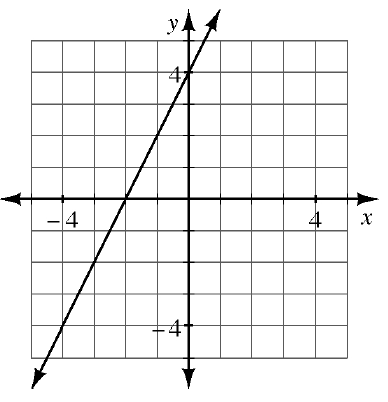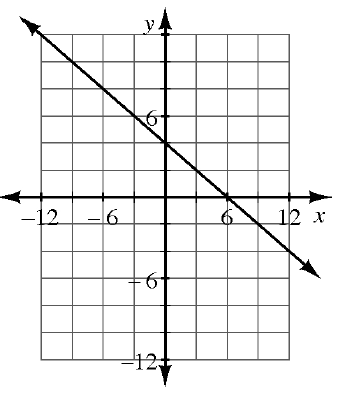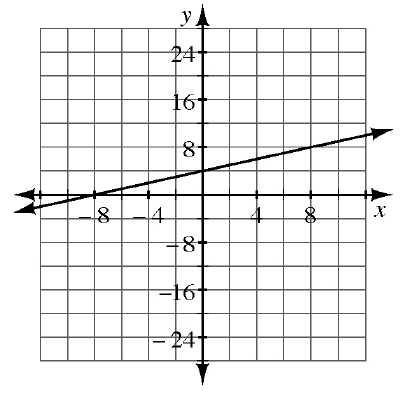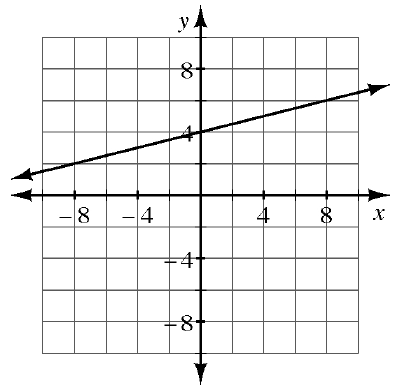### Home > GB8I > Chapter 2 Unit 2 > Lesson INT1: 2.3.1 > Problem2-98

2-98.

MATCH-A-GRAPH

Match the following graphs with their equations. Pay special attention to the scaling of each set of axes. Explain how you found each match.

1. $f(x) = \frac{1}{4}x + 4$

1. $y=\frac{1}{2}x+4$

1. $y=2x+4$

1. $f(x)=-\frac{2}{3}x+4$

1.1.1.1.Pay careful attention to the scale on each of the axes when calculating the slope of each line.

$a \rightarrow 4$
$c \rightarrow 1$